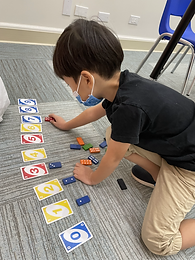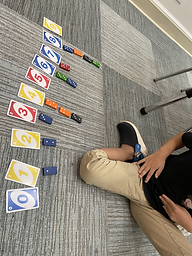## Ms. Kayla

### Target 1​

###### Lesson Type:

New

Number Operation

:

Number System

Identify the number before or after any given number 0 to 10.

###### 1:

Identify the correct number before or after a given number without having to review the entire counting sequence.

###### 2:

Given two numbers, determine which number comes in between.

Pre-K

###### Vocabulary:

Numbers, Trace, Order, Before, After, Next

Activities:

1. Students traced a set of numbers and were asked to identify each number by name. Students had difficulty identifying numbers when the numbers were out of order. Students traced over the numbers and were given the number names. After students traced the numbers, the numbers were cut out. Students put the numbers in order with the teacher's help. Students reviewed number order over and over again to complete the fill in sheet.### Home Exploration

###### Guiding Questions:## Absent Students:

### Target 2

:

###### 1:

Understand that the last number counted depicts the quantity of the objects.

Pre-K

###### Vocabulary:

Numbers, Count

Activities:

1. Students used Uno cards and domino blocks to represent numbers. Student were given a set of Uno cards 0-9 and then had to put them in order. Student were then given a bunch of dominoes and started counting the dots on them. Students matched the domino with the Uno card that showed the number that was counted. Students represented numbers 0-9 in different ways.### Home Exploration

###### Guiding Questions:### Target 3

:

###### 1:

Identify simple geometric shapes in a variety of colors, sizes, and orientations.

###### 2:

Independently identify squares, rectangles, circles, and triangles.

###### 3:

Use geometric vocabulary to define a shape (number of sides and number of corners).

###### 4:

Classify basic geometric shapes based on attributes.

Pre-K

###### Vocabulary:

Shape, Circle, Triangle, Square, Rectangle, Hexagon, Pentagon, Octagon, Sort, Color

Activities:

1. Students sorted a group of shapes using one trait (shape or color). Students identified the shapes by names as they created a shape tree.

2. Students also constructed basic geometric shapes using unifix cubes. Students constructed the outline and number of sides for each shape.### Home Exploration